Next: 2.1.1 The London Penetration Up: 2 The Characteristic Length Previous: 2 The Characteristic Length

# 2.1 The Magnetic Penetration Depth

For a static magnetic field B(0) applied parallel to a planar vacuum-to-superconducting interface, the magnetic penetration depth can be defined as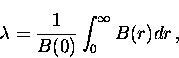(1)
where r is the distance into the superconductor measured from the surface and B(r) is a function describing the decay of the magnetic field into the superconducting region. The response of the superconductor to the magnetic field is usually defined in terms of the expectation value of the induced supercurrent density, which can be determined quantum mechanically . The momentum-space relation between the induced current and a static magnetic field for an isotropic superconductor is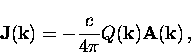(2)
where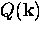is a circularly symmetric electromagnetic response kernel and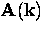is the classical vector potential. In general, the electromagnetic response kernel is a tensor. In an AC field, J, Q and A are also functions of the frequency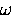--however, in this thesis only DC magnetic fields are used. In real space the relation between the induced supercurrent density and the vector potential can be written as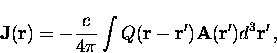(3)
where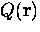is the Fourier transform of.In this case, J, Q and A are also functions of time in an AC field. The relation between Q (k) and an applied field B(0) is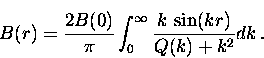(4)
Equation (2.1) and Eq. (2.4) can be combined to give a general relation for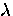in terms of Q(k)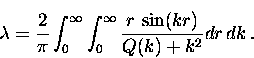(5)
Equation (2.5) is generally valid and can be used with the kernel Q (k) corresponding to any theory.Next: 2.1.1 The London Penetration Up: 2 The Characteristic Length Previous: 2 The Characteristic Length